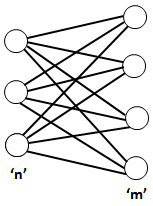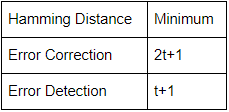## UGC NET CS 2008 Dec-Paper-2

 Question 1
The channel capacity of a band-limited Gaussian channel is given by :
 A B log2(2+S/N) B B log2(1+S/N) C B log10(1+S/N) D B loge(1+S/N)
Computer-Networks       Noisy-Channel
Question 1 Explanation:
→ Shannon Capacity (Noisy Channel) In presence of Gaussian band-limited white noise, Shannon-Hartley theorem gives the maximum data rate capacity.
C=B log2(1+S/N). where S and N are the signal and noise power, respectively, at the output of the channel.
 Question 2
The graph K3,4 has :
 A 3 edges B 4 edges C 7 edges D 12 edges
Engineering-Mathematics       Graph-Theory
Question 2 Explanation:
The graph K3,4 means it complete bipartite graph with m=4 and n=3
Total number of vertices= n + m
Total number of edges= m*n
Example:Question 3
The total number of spanning trees that can be drawn using five labelled vertices is :
 A 125 B 64 C 36 D 16
Algorithms       Minimum-Spanning-Tree
Question 3 Explanation:
To find total number of spanning trees we are using a standard formula is nn-2.
n=5
=55-2
=53
=125
 Question 4
Extremely low power dissipation and low cost per gate can be achieved in :
 A MOS ICs B C MOS ICs C TTL ICs D ECL ICs
Digital-Logic-Design       Logic-Families
Question 4 Explanation:
Emitter Coupled Logic (ECL)
The storage time is eliminated as the transistors are used in difference amplifier mode and are never driven into saturation.
1. Fastest among all logic families
2. Lowest propagation delay.
Complementary metal oxide semiconductor(CMOS)
The power dissipation is usually 10nW per gate depending upon the power supply voltage, output load etc.
1. Lowest power dissipation
2. Excellent noise immunity
3. High packing density
4. Wide range of supply voltage
5. Highest fan out among all logic families
 Question 5
An example of a universal building block is :
 A EX-OR gate B AND gate C OR gate D NOR gate
Digital-Logic-Design       Logic-Gates
Question 5 Explanation:
→ Universal building blocks are NAND and NOR gate.
→ Basic building blocks are AND,OR and NOT
 Question 6
An example of a layer that is absent in broadcast networks is :
 A Physical layer B Presentation layer C Network layer D Application layer
Computer-Networks       OSI-TCP-layers
 Question 7
The ATM cell is :
 A 48 bytes long B 53 bytes long C 64 bytes long D 69 bytes long
Computer-Networks       ATM
Question 7 Explanation:
→ The ATM cell is 53 bytes long.
→ ATM for carriage of a complete range of user traffic, including voice, data, and video signals.
→ ATM provides functionality that is similar to both circuit switching and packet switching networks.
→ ATM uses asynchronous time-division multiplexing, and encodes data into small, fixed-sized packets (ISO-OSI frames) called cells.
→ ATM uses a connection-oriented model in which a virtual circuit must be established between two endpoints before the actual data exchange begins.
 Question 8
Four jobs J1, J2, J3 and J4 are waiting to be run. Their expected run times are 9, 6, 3 and 5 respectively. In order to minimise average response time, the jobs should be run in the order:
 A J1 J2 J3 J4 B J4 J3 J2 J1 C J3 J4 J1 J2 D J3 J4 J2 J1
Operating-Systems       Process-Scheduling
Question 8 Explanation:
Since arrival time is not given assume arrival time of each process as"0" Option (A):Question 9
Suppose it takes 100 ns to access page table and 20 ns to access associative memory. If the average access time is 28 ns, the corresponding hit rate is :
 A 100 percent B 90 percent C 80 percent D 70 percent
Operating-Systems       Memory-Management
Question 9 Explanation:
Given data,
-- Access page table time=100 ns
-- Associate memory=20 ns
-- hit ratio=X
-- Miss ratio=1-X
-- Average Access Time=28
Step-1: AAT= Hit Ratio*Access page table + Miss Ratio*Associate memory
= X*20 + (1-X*100) [Note: If X=0.9 then we are getting exact AAT]
= 28 ns
(or)
28=X*20+(1-X*100)
X=0.9
 Question 10
Transmission of N signals, each band limited to fm Hz by TDM, requires a minimum band-width of :
 A fm B 2 fm C N fm D 2N fm
Computer-Networks       Access-Control-Methods
Question 10 Explanation:
→ Time-division multiplexing (TDM) is a digital process that allows several connections to share the high bandwidth of a link Instead of sharing a portion of the bandwidth as in FDM, time is shared. Each connection occupies a portion of time in the link.
→ Since here we have N signals and each signal requires frequency band of fm Hz then total bandwidth required is Nfm Hz.
 Question 11
If a code is ‘t’ error detecting, the minimum hamming distance should be equal to :
 A t-1 B t C t+1 D 2t+1
Digital-Logic-Design       Number-Systems
Question 11 Explanation:Question 12
A relation R in {1, 2, 3, 4, 5, 6} is given by { (1, 2), (2, 3), (3, 4), (4, 4), (4, 5) }. This relation is :
 A reflexive B symmetric C transitive D not reflexive, not symmetric and not transitive
Engineering-Mathematics       Set-Theory
Question 12 Explanation:
Reflexive: A relation R is said to be reflexive if for all elements of a set x belongs to R (x,x) exists.
Since in above question {(1,1), (2,2),(3,3),(4,4),(5,5),(6,6)} does not exist in given relation so it is not reflexive.
Symmetric: A relation R is symmetric if (x,y) belong to R then (y,x) must belong to R. Since in above question (1,2) exists but (2,1) does not exist in given relation so given relation is not symmetric.
Transitive: A relation is transitive if (x,y) belongs to R and (y,z) belongs to R then (x,z) must belongs to R.
In above question (1, 2), (2, 3) exist in given relation but (1,3) does not exist there so given relation is not transitive.
Hence correct option is option(D)
There are 12 questions to complete.

Register Now